# How do you train a hMM model in practice ?

The joint probability distribution for the HMM model is given by the following equation where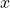are the observed data points and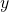the corresponding latent states: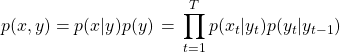Before proceeding to answer the question on training a HMM, it makes sense to ask following questions

1. What is the problem in hand for which we are training the above hidden Markov Model. Notice that the above model is generic and can be applied to any problem
2. Once we know what problem we are solving using the above model, we need to know if we have labelled data.
1. If we have labelled data: Note that while HMM is a latent variable model, in some cases it is possible to have labelled data, for popular problems such as POS tagging. If we have labelled data (with state labels given to us with observed emissions), we can use counting based heuristics to estimate the transition and emission probabilities using MLE. For instance, in the POS tagging case, the transition probabilities can be computed by how many times we transition from one tag to another, while the emission probabilities can be computed by the ratio #of times we observe a specific word given a tag/# of words with the tag.
2. If we don’t have labelled data, then it becomes an unsupervised problem and we need to use EM algorithm to estimate the transition and emission probabilities.
1. So for example, if the problem is to predict the PoS tags given a sequence and assume the data is given(not labelled), we use EM algorithm as follows
2. In E step, we assume some posterior probabilities to begin with.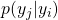is estimated using maximum likelihood estimator and comes out to be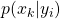are also estimated using MLE and comes out as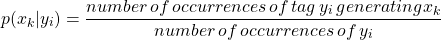Initializeand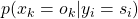3. M step is used to update the probabilities/parameters of the model using MLE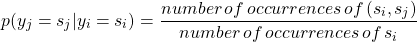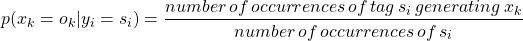3. When we have some labeled data, we can get an initial estimate using MLE on the labelled data (the counting technique in 1), but can refine it with EM by augmenting with the unlabelled data.